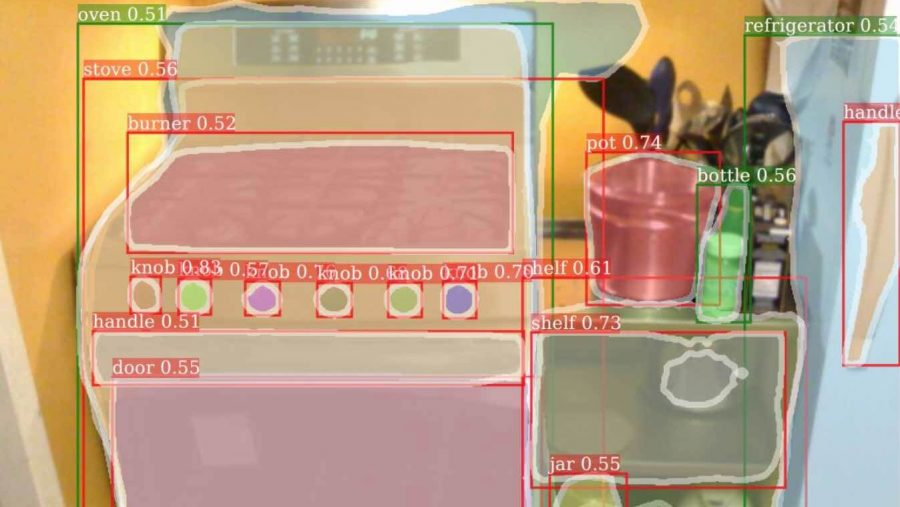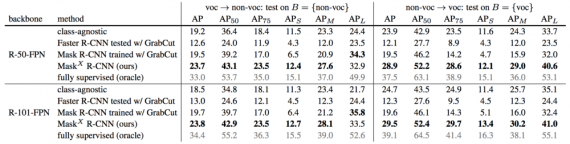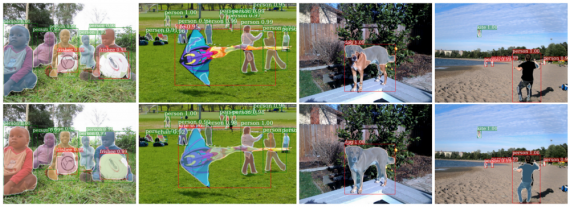• # FAIR Proposed a New Partially Supervised Trading Paradigm to Segment Every ThingObject detectors have become significantly more accurate and gained new capabilities. One of the most exciting is the ability to predict a foreground segmentation mask for each detected object, a task called instance segmentation. In practice, typical instance segmentation systems are restricted to a narrow slice of the vast visual world that includes only around 100 object categories. A principal reason for this limitation is that state-of-the-art instance segmentation algorithms require strong supervision and such supervision may be limited and expensive to collect for new categories. By comparison, bounding box annotations are more abundant and cheaper.

FAIR (Facebook AI Research) introduced a new partially supervised instance segmentation task and proposed a novel transfer learning method to address it. The partially supervised instance segmentation task as follows:

1. Given a set of categories of interest, a small subset has instance mask annotations, while the other categories have only bounding box annotations.
2. The instance segmentation algorithm should utilize this data to fit a model that can segment instances of all object categories in the set of interest.

Since the training data is a mixture of strongly annotated examples (those with masks) and weakly annotated examples (those with only boxes), the task is referred to partially supervised. To address partially supervised instance segmentation, a novel transfer learning approach built on Mask R-CNN. Mask R-CNN is well-suited to this task because it decomposes the instance segmentation problem into the subtasks of bounding box object detection and masks prediction.

## Learning to Segment Every Thing

Let C be the set of object categories for which instance segmentation model is trained. All training examples in C are annotated with instance masks. It is to be assumed that C = A ∪ B where samples from the categories in A have masks, while those in B have only bounding boxes. Since the examples of the B categories are weakly labeled w.r.t., the target task (instance segmentation), it is referred to train on the combination of strong and weak labels as a partially supervised learning problem. Given an instance segmentation model like Mask RCNN that has a bounding box detection component and a mask prediction component, a model Mask^X RCNNmethod that transfers category-specific information from the model’s bounding box detectors to its instance mask predictors.

This method is built on Mask R-CNN, because it is a simple instance segmentation model that also achieves state-of-the-art results. In Mask R-CNN, the last layer in the bounding box branch and the last layer in the mask branch both contain category-specific parameters that are used to perform bounding box classification and instance mask prediction, respectively, for each category. Instead of learning the category-specific bounding box parameters and mask parameters independently, authors propose to predict a category’s mask parameters from its bounding box parameters using a generic, category-agnostic weight transfer function that can be jointly trained as part of the whole model.

For a given category c, let w(det) be the class-specific object detection weights in the last layer of the bounding box head, and w(seg) be the class-specific mask weights in the mask branch. Instead of treating w(seg) as model parameters, w(seg) is parameterized using a generic weight prediction function T (·):

where θ are class-agnostic, learned parameters. The same transfer function T(·) may be applied to any category c and, thus, θ should be set such that Tgeneralizes to classes whose masks are not observed during training.

T (·) can be implemented as a small fully connected neural network. Figure 1 illustrates how the weight transfer function fits into Mask R-CNN to form Mask^X R-CNN. Note that the bounding box head contains two types of detection weights: the RoI classification weights w(cls) and the bounding box regression weights w(box).

## Experiments on COCO

This method is evaluated on COCO dataset which is small-scale w.r.t. the number of categories but contains exhaustive mask annotations for 80 categories. This property enables rigorous quantitative evaluation using standard detection metrics, like average precision (AP). Each class has a 1024-d RoI classification parameter vector w(cls) and a 4096- d bounding box regression parameter vector w(box) in the detection head, and a 256-d segmentation parameter vector w(seg) in the mask head. The output mask resolution is M × M = 28 × 28. Table below compares full Mask^X R-CNN method (i.e., Mask R-CNN with ‘transfer+MLP’ and T implemented as ‘cls+box, 2-layer, LeakyReLU’) and the class-agnostic baseline using end-to-end training.Mask^X R-CNN outperforms these approaches by a large margin (over 20% relative increase in mask AP).

## ResultMask predictions from the class-agnostic baseline (top row) vs. Mask^X R-CNN approach (bottom row). Green boxes are classes in set A while the red boxes are classes in set B. The left 2 columns are A = {voc} and the right 2 columns are A = {non-voc}.

This research addresses the problem of large-scale instance segmentation by formulating a partially supervised learning paradigm in which only a subset of classes have instance masks during training while the rest have box annotations.The FAIR proposes a novel transfer learning approach, where a learned weight transfer function predicts how each class should be segmented based on parameters learned for detecting bounding boxes. Experimental results on the COCO dataset demonstrate that this method significantly improves the generalization of mask prediction to categories without mask training data. This model will help to build a large-scale instance segmentation model over 3000 classes in the Visual Genome dataset.